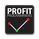# Trend Scores + Volume-Weighted Trend Scores

730 visningar
730
Here is a simple indicator based on Tushar Chande's TrendScore .

The main purpose of the TrendScore is to determine the strength and direction of a trend, which it does by comparing the current price to the prices within a user-defined window of historical prices.

In the input menu, the user defines the starting and ending period. The current price is then compared to each historical price. If the current price is greater than the given historical price, then the TrendScore is incremented, while it is decremented if it is below the given historical price. TrendScore values fluctuate between a maximum of 100 and a minimum of -100, with 100 meaning that the current price is greater than each historical price in the window and a value of -100 meaning the inverse is true.

We then use the same process to calculate the volume trend score by passing in volume to the 'getTrendScore' function. Lastly, the indicator also also calculates a 'volume-weighted trend score'. This is simply the average of the price trend score and the volume trend score. It is not plotted by default, but users can set the input option to true in the input menu and it will be plotted as a yellow line (as seen in the bottom chart).

The Chart:
The trend scores for price are plotted as a histogram. We've summarized the meaning behind its color changes below:
-If ( trendScore == 100)
then color = dark green

-if ( trendScore < 100 and trendScore is increasing)
then color = light green

-if ( trendScore > 0 and trendScore is DECREASING)
then color = pink

-if ( trendScore < 0)
then color = red

The volume trend score is plotted as a blue line. We felt that using a similar coloring system for the volume trend scores would over-crowd the chart and take away from the simplicity that makes this indicator useful. The volume-weighted trend score is plotted as a yellow line.

The main price bars change color based on the price trend score to make the values easier to visualize as well.

Interpretation:
This is a pretty versatile indicator. We summarized the ways in which traders can use it:
-Enter Long Positions when the trend score crosses zero from negative to positive territory.

-Exit Long Positions when the trend score was previously 100 and begins decreasing (ie bar color changes from dark green to pink).

-Spot bearish divergences when price trend score is 100 or relatively high and the volume trend score decreases significantly.

-Identify bullish divergences when price trend score is relatively low and volume trend score is increasing.